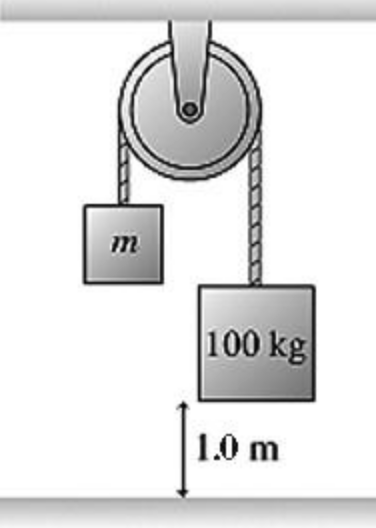In: Physics

# The figure shows a 100-kg block being released from rest from a height of 1.0 m.

The figure shows a 100-kg block being released from rest from a height of 1.0 m. It then takes it 0.90 s to reach the floor. What is the mass of the other block? The pulley has no appreciable mass or friction.A. 60 kg B. 48 kg C. 54 kg D. 42 kg
The figure shows a 100 - kg block being released from rest from a height of 1.0 m. It then takes it 0.90 s to reach the floor. What is the mass of the other block? The pulley has no appreciable mass or friction. A. 60 kg B. 48 kg C. 54 kg D. 42 kg

## Solutions

##### Expert Solution

Concepts and reason

The concepts required to solve this problem are Newton's second law and kinematics equation of motion. First, use the kinematics equation of motion to solve for the acceleration of the blocks. Finally, use Newton's second law for both the blocks to solve for the mass of the other block.

Fundamentals

Newton's second law states that the net force on an object is the product of the object's mass and the final acceleration of the object. The expression of newton's second law is, $$\sum F=m a$$

Here, $$\sum F$$ is the sum of all the forces on the object, $$m$$ is mass of the object, and $$a$$ is the acceleration of the object. The kinematics equation of motion is, $$y=v_{\mathrm{i}} t+\frac{1}{2} a_{y} t^{2}$$

Here, $$y$$ is the distance travelled by the object, $$v_{\mathrm{i}}$$ is the initial velocity, $$t$$ is the time taken, $$a_{y}$$ is the acceleration along $$y$$ -axis. The weight of a block is, $$w=m g$$

Here, $$m$$ is the mass, and $$g$$ is the acceleration due to gravity.

Rearrange the kinematics equation to solve for acceleration.

$$\begin{array}{c} y=v_{\mathrm{i}} t+\frac{1}{2} a_{y} t^{2} \\ \frac{1}{2} a_{y} t^{2}=y-v_{\mathrm{i}} t \\ a_{y}=\frac{2\left(y-v_{\mathrm{i}} t\right)}{t^{2}} \end{array}$$

Substitute $$0 \mathrm{~m} / \mathrm{s}$$ for $$v_{\mathrm{i}}, 0.90 \mathrm{~s}$$ for $$t$$, and $$1.0 \mathrm{~m}$$ for $$y$$ in the equation $$a_{y}=\frac{2\left(y-v_{\mathrm{i}} t\right)}{t^{2}}$$ and calculate the acceleration of the blocks.

\begin{aligned} a_{y} &=\frac{2(1.0 \mathrm{~m}-(0)(0.9 \mathrm{~s}))}{(0.9 \mathrm{~s})^{2}} \\ &=2.469 \mathrm{~m} / \mathrm{s}^{2} \end{aligned}

The blocks start from rest, so the initial velocity is zero. The distance the block moves down is the height of the heavier block 1.0min time $$0.90$$ s. Substitute the values in the rearranged kinematics equation of motion to calculate the acceleration.

Use the Newton's second law along $$y$$ direction. Substitute $$T-m g$$ for $$\sum F_{y}$$ in the equation $$\sum F_{y}=m a_{y}$$ and solve for Tension.

$$\begin{array}{l} T-m g=m a_{y} \\ T=m g+m a_{y} \end{array}$$

Here, $$T$$ is tension in the rope, $$m g$$ is weight of the block and $$g$$ is the acceleration due to gravity. Substitute $$M g-T$$ for $$\sum F_{y}$$ in the above equation $$\sum F_{y}=M a_{y}$$. $$M g-T=M a_{y}$$

Here, $$M$$ is the mass of heavier block. Substitute $$m g+m a_{y}$$ for $$T$$ in the equation $$M g-T=M a_{y}$$ and solve for $$m$$.

$$\begin{array}{c} M g-\left(m g+m a_{y}\right)=M a_{y} \\ m\left(g+a_{y}\right)=M\left(g-a_{y}\right) \\ m=\frac{M\left(g-a_{y}\right)}{\left(g+a_{y}\right)} \end{array}$$

Substitute $$100 \mathrm{~kg}$$ for $$M, 9.81 \mathrm{~m} / \mathrm{s}^{2}$$ for $$g$$, and $$2.469 \mathrm{~m} / \mathrm{s}^{2}$$ for $$a_{y}$$ in the equation $$m=\frac{M\left(g-a_{y}\right)}{\left(g+a_{y}\right)}$$ and calculate $$m$$.

$$\begin{array}{c} m=\frac{(100 \mathrm{~kg})\left(9.81 \mathrm{~m} / \mathrm{s}^{2}-2.469 \mathrm{~m} / \mathrm{s}^{2}\right)}{\left(9.81 \mathrm{~m} / \mathrm{s}^{2}+2.469 \mathrm{~m} / \mathrm{s}^{2}\right)} \\ =59.78 \mathrm{~kg} \\ \approx 60 \mathrm{~kg} \end{array}$$

The Newton's second law along $$y$$ direction is, $$\sum F_{y}=m a_{y}$$

Here, $$\sum F_{y}$$ is sum of all the force in $$y$$ direction, and $$a_{y}$$ is the $$y$$ component of acceleration. The acceleration of both the blocks is the same as there is no friction, and they are connected with string. Two forces act on both the blocks. The tension $$T$$ in an upward direction and weight in a downward direction. The motion of the other block is in an upward direction so tension is in the direction of motion so positive and weight will be opposite to acceleration so negative. The motion of the heavier block is in a downward direction so tension is opposite to the direction of motion or acceleration so negative and weight $$M g$$ is in the direction of acceleration so positive.

The mass of the other block is 60kg.